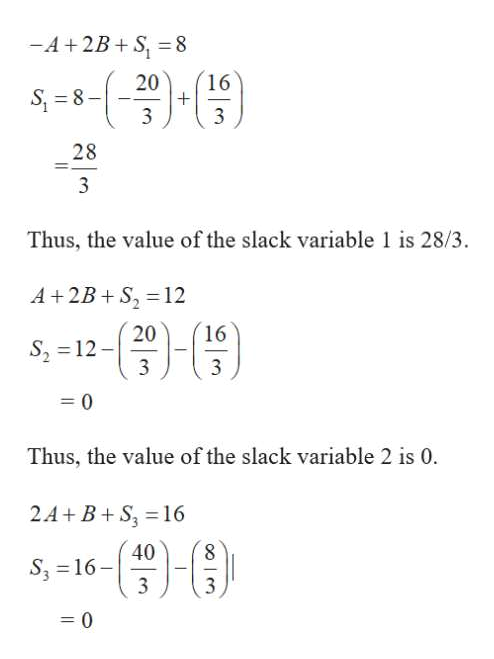# 19. Given the linear program  Max 3A +4B  s.t.-1A + 2B< = 8 1A + 2B< =12 2A + 1B< =16       A,B< = 0d. What is the value of slack variable 1?e. What is the value of slack variable 2? f. What is the value of slack variable 3?

Question
18 views

19. Given the linear program
Max 3A +4B
s.t.
-1A + 2B< = 8
1A + 2B< =12
2A + 1B< =16
A,B< = 0

d. What is the value of slack variable 1?
e. What is the value of slack variable 2?
f. What is the value of slack variable 3?

check_circle

Step 1

The given LPP is as follows.

Z (A, B) = 3A +4B +0S1+0S2+0S3.

Subject to its constraints,

–1A + 2B + S1 = 8                                                       …… (1)

1A + 2B+         S2=12                                                 …… (2)

2A + 1B              + S3 =16                                          …… (3)

A, B >= 0

Solve L1 and L2 as follows,

Step 2

Substitute the value of A and B in the given three equat...help_outlineImage Transcriptionclose-A+2B+S, = 8 20 16 S 8 3 3 28 3 Thus, the value of the slack variable 1 is 28/3. A+2B S2 12 16 20 S, 12- 3 3 0 Thus, the value of the slack variable 2 is 0. 2A+ B+S 16 40 8 S3 16 3 0 fullscreen

### Want to see the full answer?

See Solution

#### Want to see this answer and more?

Solutions are written by subject experts who are available 24/7. Questions are typically answered within 1 hour.*

See Solution
*Response times may vary by subject and question.
Tagged in

### Math